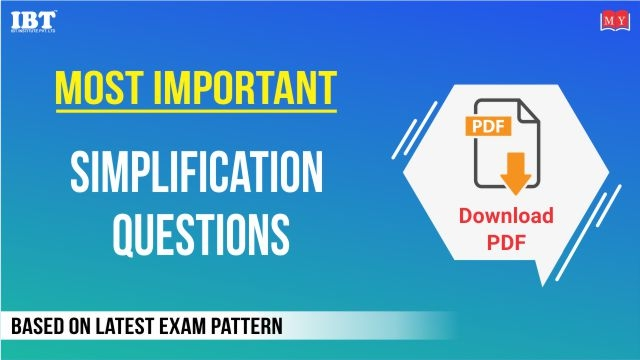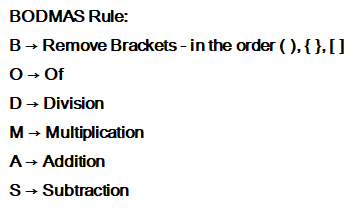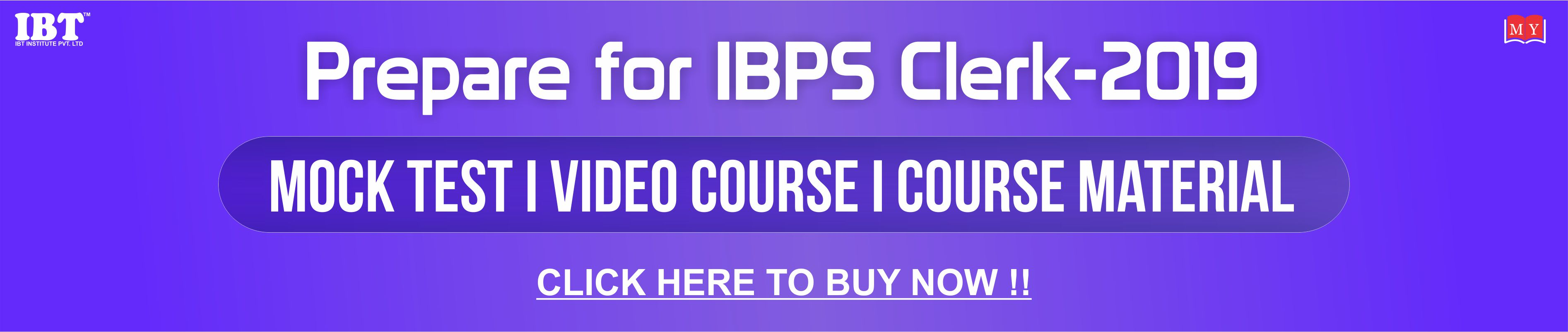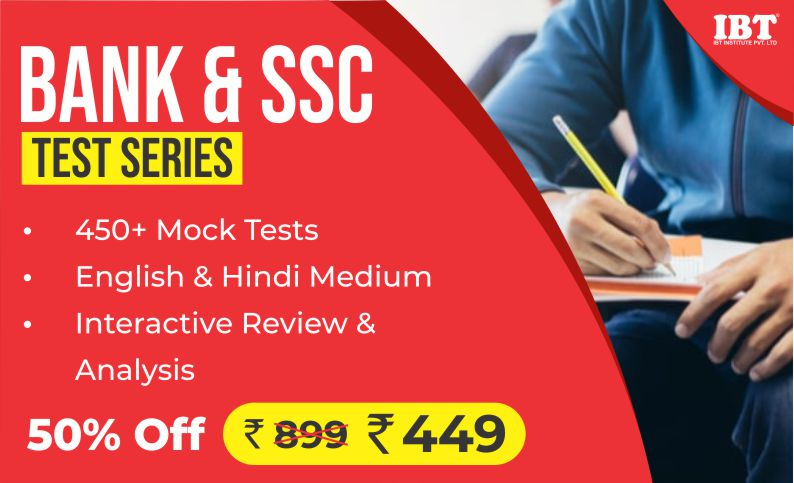Most Important Simplifications 100 Questions PDF for IBPS Clerk exam 2019, Download PDF with Solution# Most Important Simplifications 100 Questions PDF for IBPS Clerk exam 2019, Download PDF with solution

Dear Students,

IBPS Clerk Exam 2019 is going to be conducted, We hope you all have been preparing well for this exam. To brush up your preparation at this stage, here are some most important questions for IBPS Clerk Prelims Exam 2019. We have shared a Free PDF of Most Important Simplifications 100 questions PDF for IBPS Clerk exam 2019 along with their Solution. You Should attempt these Top 100 important Questions in order to crack the upcoming IBPS Clerk Exam 2019.

Smart Tips to Solve Simplification
Simplification is a topic that is the easiest to solve in quant’s section and at least 5 questions in every banking examination are asked from this part. So, one can easily grab 5-6 marks in Quantitative Aptitude Section if he/she is good at calculations. All that you need to do for this topic is to be fast with calculations and keep practicing, it will always stand you in good stead. The important trick to deal with this section is to remember the BODMAS Rule and have all the tables, cubes, squares entirely grasped.
Use following rule to evaluate expression if it comprises of various symbols.Simplification comprises of various topics like fractions, surds and indices and squares and cubes.

1. Squares and cubes
Best method to solve these questions by memorizing the squares and cubes of first 50 and 20 numbers respectively.
Trick to find the Square of a numbers Ending in 5
452= 1225
1) Multiply the first digit by the first digit plus one:- 4 * ( 4 + 1 ) = 20
2) Write the number 25 (square of 5) next to the result from Step 1

Ans:- 2025

2. Approximation question
Find the value of following expression:- 441*20+341*29.9
a)111.35.   b) 252.25    c) 133.45    d) None of this

Solution:
We can write given expression as,
440 x 20+ 340 x 30
= 8800+10200
=19000
19000 is not the actual answer. The actual answer is 19015.9. So we have reached very close to the correct answer in almost no time. This is a small calculated risk that you should be ready to take in the exam to save time.

Note : Other questions from topics fractions and surds and indices can be easily solved by using above methods and LCM method.